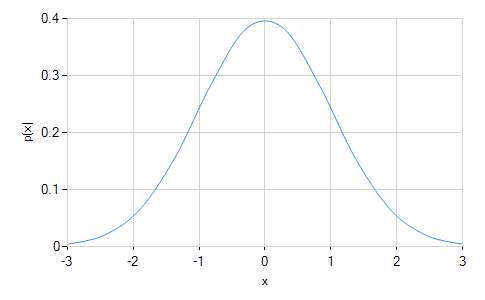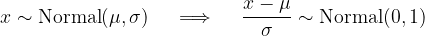﻿NormalDistribution Class# NormalDistribution Class

Represents a normal (Gaussian) distribution.Inheritance Hierarchy

Namespace:  Meta.Numerics.Statistics.Distributions
Assembly:  Meta.Numerics (in Meta.Numerics.dll) Version: 4.1.4Syntax
`public sealed class NormalDistribution : ContinuousDistribution`

The NormalDistribution type exposes the following members.Constructors
NameDescriptionNormalDistribution
Initializes a new standard normal distribution.NormalDistribution(Double, Double)
Initializes a new normal distribution with the given mean and standard deviation.
TopProperties
NameDescriptionExcessKurtosis
Gets the excess kurtosis of the distribution.
(Overrides UnivariateDistributionExcessKurtosis.)Mean
Gets the mean of the distribution.
(Overrides UnivariateDistributionMean.)Median
Gets the median of the distribution.
(Overrides ContinuousDistributionMedian.)Skewness
Gets the skewness of the distribution.
(Overrides UnivariateDistributionSkewness.)StandardDeviation
Gets the standard deviation of the distribution.
(Overrides UnivariateDistributionStandardDeviation.)Support
Gets the interval over which the distribution is non-vanishing.
(Inherited from ContinuousDistribution.)Variance
Gets the variance of the distribution.
(Inherited from UnivariateDistribution.)
TopMethods
NameDescriptionCentralMoment
Computes a central moment of the distribution.
(Overrides ContinuousDistributionCentralMoment(Int32).)Cumulant
Computes a cumulant of the distribution.
(Overrides UnivariateDistributionCumulant(Int32).)Equals
Determines whether the specified object is equal to the current object.
(Inherited from Object.)ExpectationValue
Computes the expectation value of the given function.
(Inherited from ContinuousDistribution.)FitToSample
Computes the normal distribution that best fits the given sample.GetHashCode
Serves as the default hash function.
(Inherited from Object.)GetRandomValue
Generates a random variate.
(Overrides ContinuousDistributionGetRandomValue(Random).)GetRandomValues
Generates the given number of random variates.
(Inherited from ContinuousDistribution.)GetType
Gets the Type of the current instance.
(Inherited from Object.)Hazard
Computes the hazard function.
(Inherited from ContinuousDistribution.)InverseLeftProbability
Returns the point at which the cumulative distribution function attains a given value.
(Overrides ContinuousDistributionInverseLeftProbability(Double).)InverseRightProbability
Returns the point at which the right probability function attains the given value.
(Overrides ContinuousDistributionInverseRightProbability(Double).)LeftProbability
Returns the cumulative probability to the left of (below) the given point.
(Overrides ContinuousDistributionLeftProbability(Double).)ProbabilityDensity
Returns the probability density at the given point.
(Overrides ContinuousDistributionProbabilityDensity(Double).)RawMoment
Computes a raw moment of the distribution.
(Overrides ContinuousDistributionRawMoment(Int32).)RightProbability
Returns the cumulative probability to the right of (above) the given point.
(Overrides ContinuousDistributionRightProbability(Double).)ToString
Returns a string that represents the current object.
(Inherited from Object.)
TopRemarks

A normal distribution is a bell-shaped curve centered at its mean and falling off symmetrically on each side. It is a two-parameter distribution determined by giving its mean and standard deviation, i.e. its center and width. Its range is the entire real number line, but the tails, i.e. points more than a few standard deviations from the means, fall off extremely rapidly.A normal distribution with mean zero and standard deviation one is called a standard normal distribution. Any normal distribution can be converted to a standard normal distribution by re-parameterizing the data in terms of "standard deviations from the mean", i.e. z = (x - μ) / σ.Normal distribution appear in many contexts. In practical work, the normal distribution is often used as a crude model for the distribution of any continuous parameter that tends to cluster near its average, for example human height and weight. In more refined theoretical work, the normal distribution often emerges as a limiting distribution. For example, it can be shown that, if a large number of errors affect a measurement, then for nearly any underlying distribution of error terms, the distribution of total error tends toward a normal distribution.

The normal distribution is often called a Gaussian, after the mathematician Friedrich Gauss.See Also